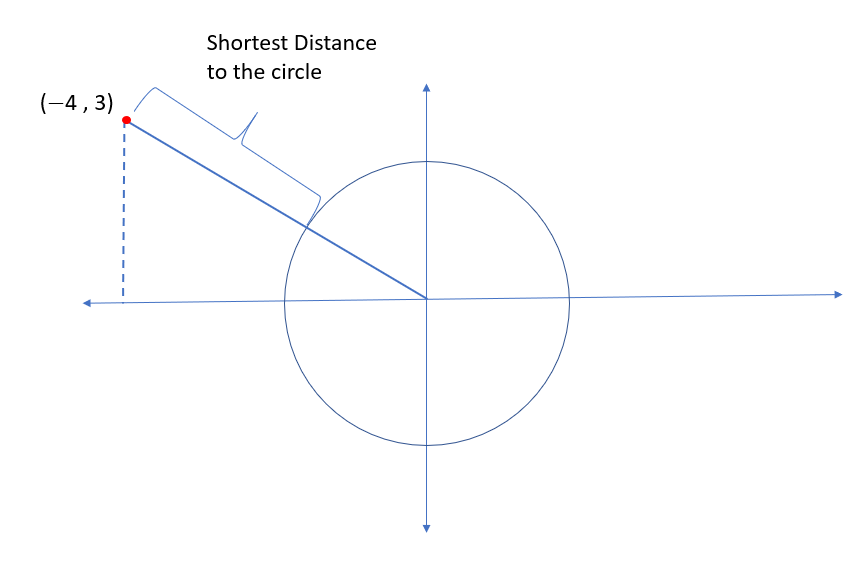# IPMAT Question Paper 2020 | IPM Indore Quants

###### IPMAT Sample Paper | IPMAT Question Paper | Question 2

IPMAT 2020 Question Paper IPM Indore Quantitative Ability. Solve questions from IPMAT 2020 Question Paper from IPM Indore and check the solutions to get adequate practice. The best way to ace IPMAT is by solving IPMAT Question Paper. To solve other IPMAT Sample papers, go here: IPM Sample Paper

Question 2 : The shortest distance from the point (-4,3) to the circle x2 + y2 = 1 is __________.

## Best CAT Coaching in Chennai

#### CAT Coaching in Chennai - CAT 2022Limited Seats Available - Register Now!From the above diagram, we can see that, we can draw many lines to the circle that can be a secant or a tangent. We need to find the line which gives us the shortest distance of the point from the circle.

It is very evident that the line joining the center of the cirle to the point (-4,3) will give us the shortest distance.

As we can see from the diagram, we have a right triangle with base length as 4 and height as 3. Therefore the distance of the point (-4,3) from the center of the circle is the hypotenuse and its length is 5. (3,4,5 is a pythagorean triplet)

Therefore, the distance of the point (-4,3) from the circle = 5 - radius of the circle = 5 - 1 = 4

The question is " The shortest distance from the point (-4,3) to the circle x2 + y2 = 1 is __________. "

##### Where is 2IIM located?

2IIM Online CAT Coaching
A Fermat Education Initiative,
58/16, Indira Gandhi Street,
Kaveri Rangan Nagar, Saligramam, Chennai 600 093

##### How to reach 2IIM?

Mobile: (91) 99626 48484 / 94459 38484
WhatsApp: WhatsApp Now
Email: info@2iim.com